• Call Now

1800-102-2727•

# Geometric Tools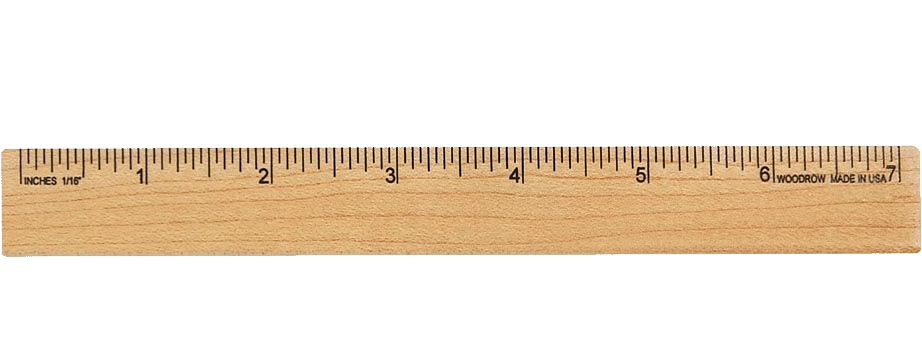A ruler, often known as a straightedge, is used to draw straight lines and measure line segment lengths. A ruler, also known as a line gauge, is a mathematical instrument used in geometry and a variety of engineering applications. It's a straight edge that was originally solely used to create straight lines. It may also determine the object's distance. It is graded in centimeters and millimeters on one side and inches on the other for our convenience. It may be used to measure lengths in both metric and customary units. The “Hash Markings” are the intervals or marks specified in the ruler.

## Compass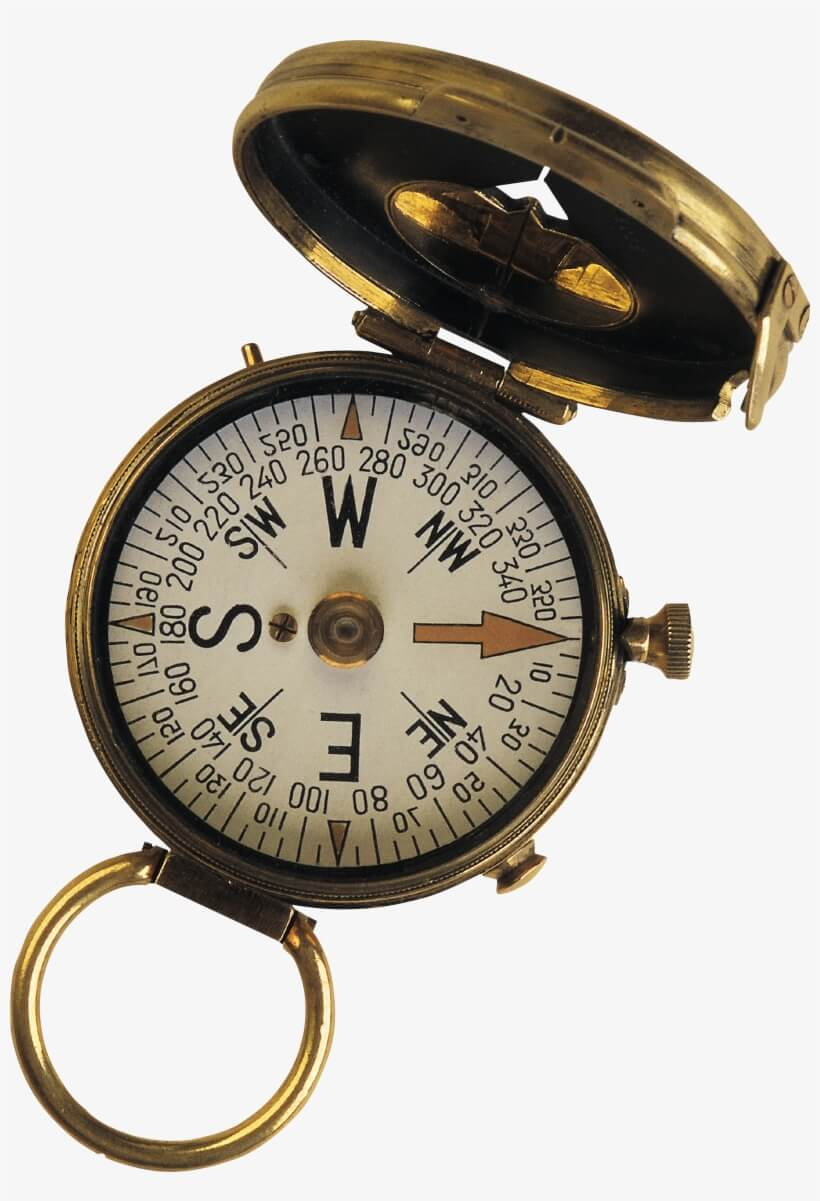It's a V-shaped gadget with a pointer on one side and a pencil on the other. It's possible to change the distance between the pencil and the pointer. Trace arcs, circles, and angles using it. It's also used to indicate equal lengths. A compass is a mathematical instrument that is used to design geometrical figures like circles. It's also used for the intersection of line segments and tools, which are used to intersect line segments and aid in the discovery of the forms' midpoints.

## Mathematical protractor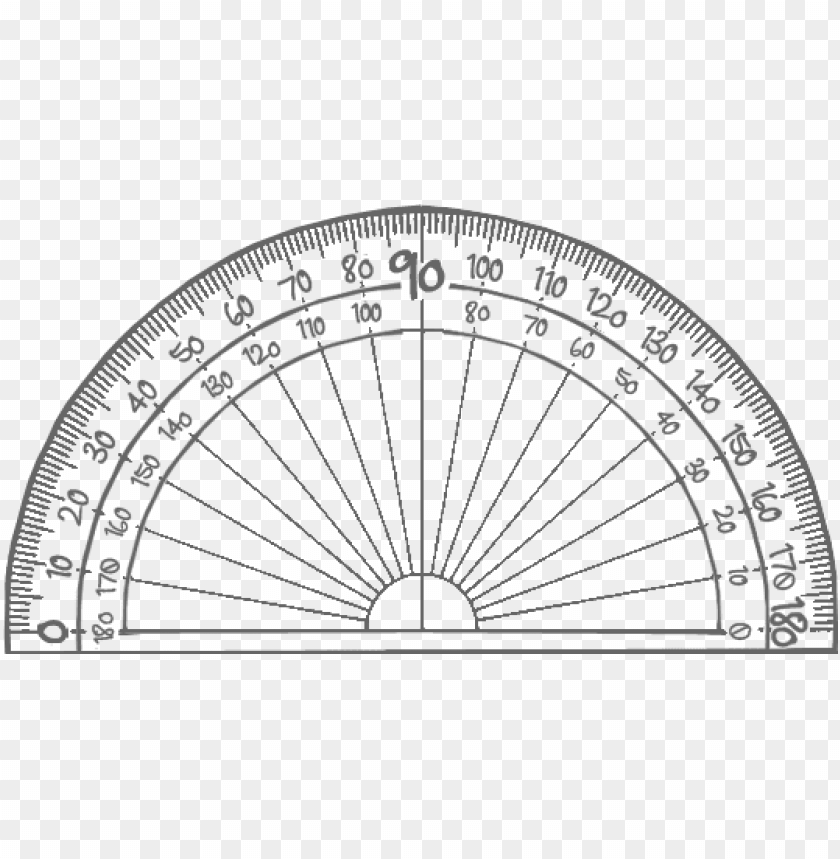It's a semi-circular disc that's used to measure and draw angles. It has a graded range of 0 to 180 degrees and may be used to measure any angle that falls within that range. It contains two sets of marks, one from left to right and the other from right to left. The protractor's inner and outer readings complement each other. It indicates that the sum of the inner and outside readings equals 180 degrees.

## Divider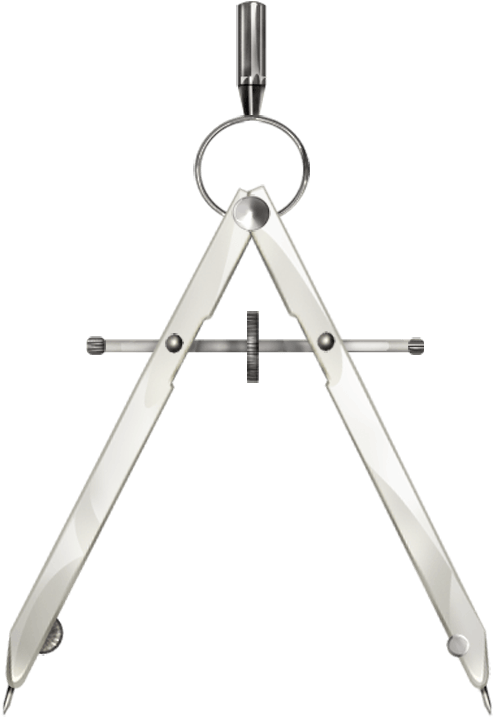With its ‘V'-shaped structure, it resembles a compass. On both ends of the ‘V,' however, there are points. It's used to measure and compare lengths since the distance between them may be adjusted.

To mark the distance or designate divisions, the terms divider and compass are frequently employed. The main distinction between a compass and a divider is that a compass is a drafting device with one sharp point in the centre and a pen or pencil on the other point to delineate the circle or other functions. The divider, on the other hand, has two sharp points, one of which serves as a centre and the other as a marking tool.

## Set-Squares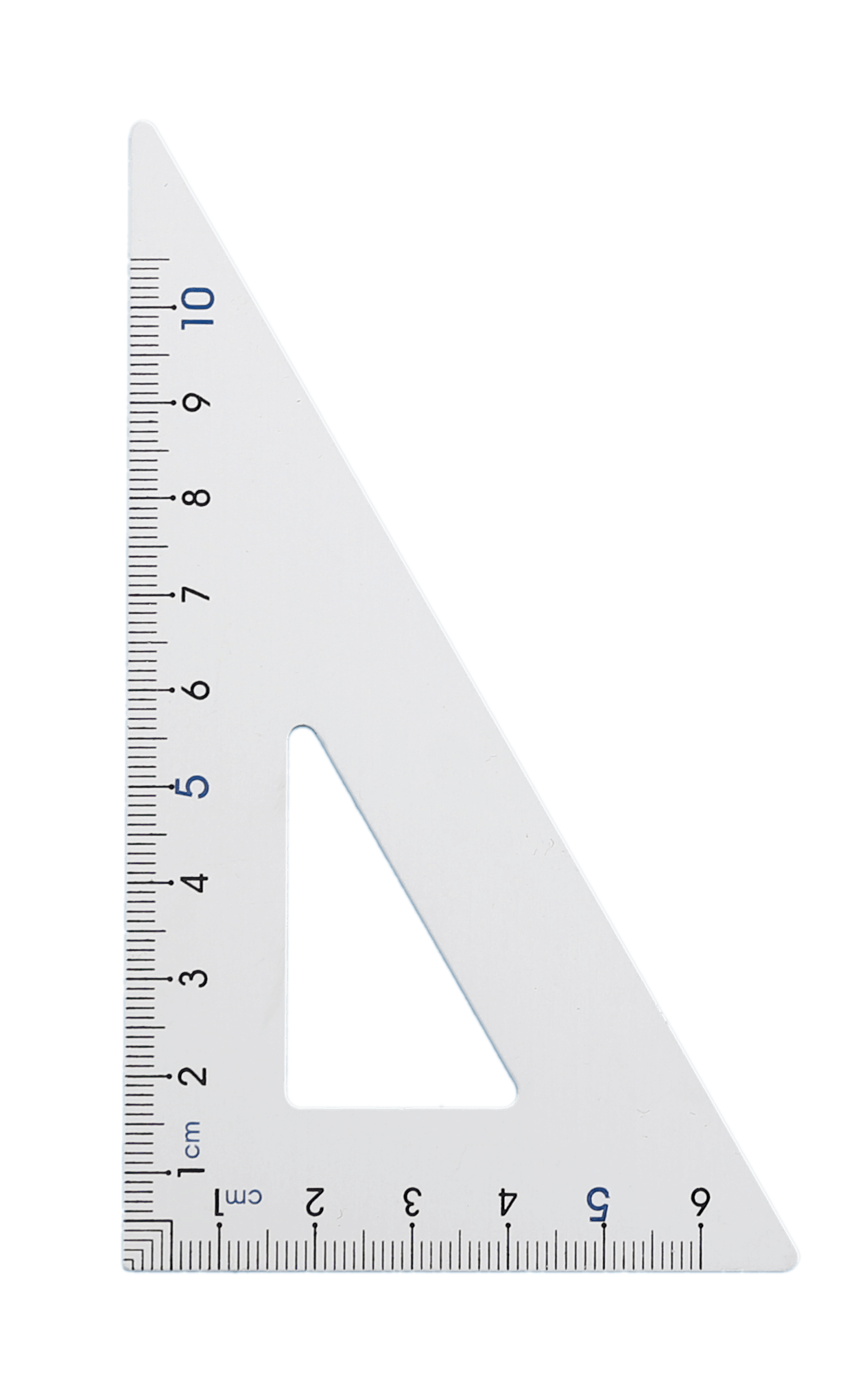Set squares are another mathematics tool that is often seen in a geometry box. These are the triangle plastic pieces that have had a part of the space between them eliminated. Set squares are offered in two varieties on the market. One has a 45-degree angle, while the other has a 30-60-degree angle. The 45-degree set square has a 90-degree angle, whereas the 30-degree and 60-degree set squares have a right angle. Vertical lines are drawn using the 45 degrees set square. Set squares can be used to create parallel lines, perpendicular lines, and standard angles, among other thingsTalk to our expert
Resend OTP Timer =
By submitting up, I agree to receive all the Whatsapp communication on my registered number and Aakash terms and conditions and privacy policy